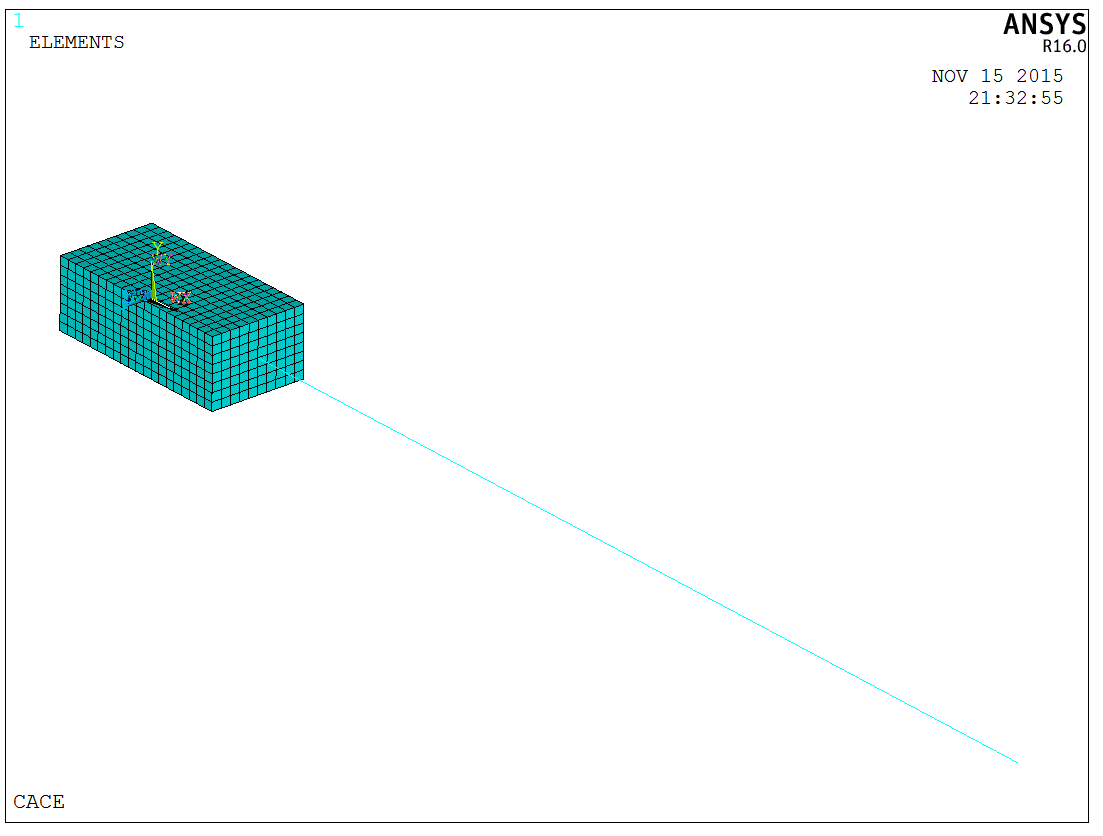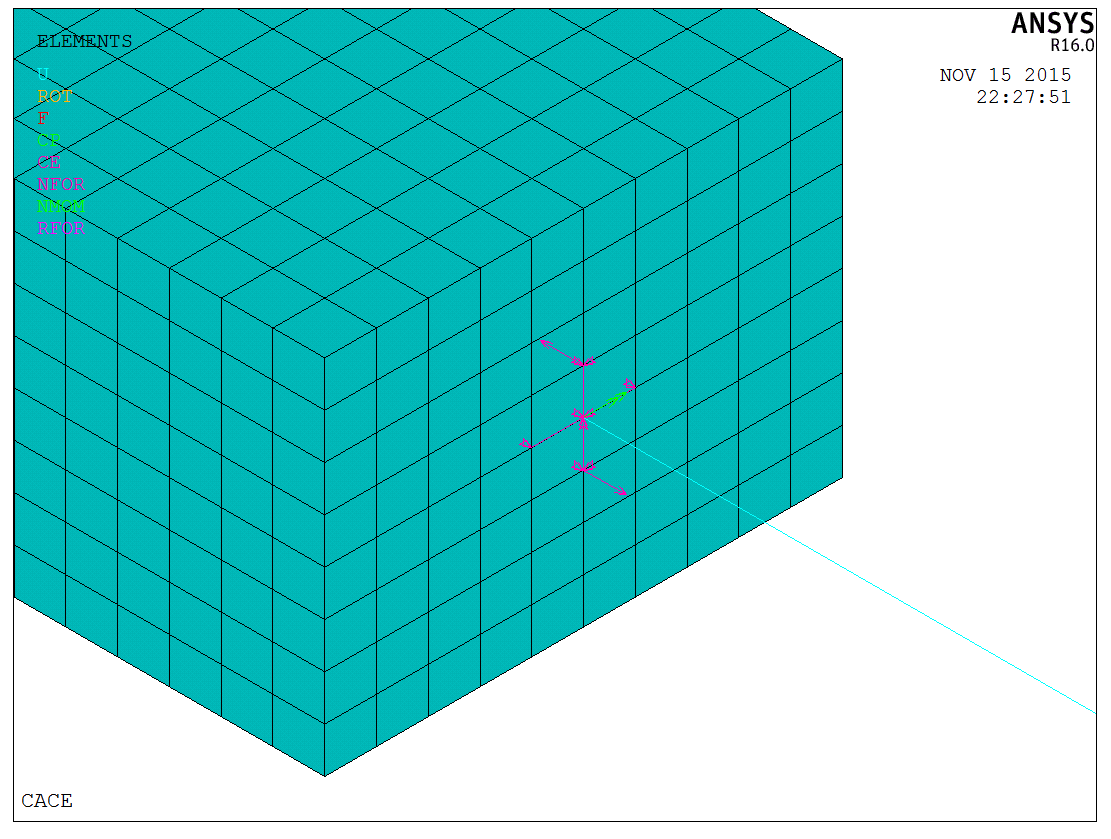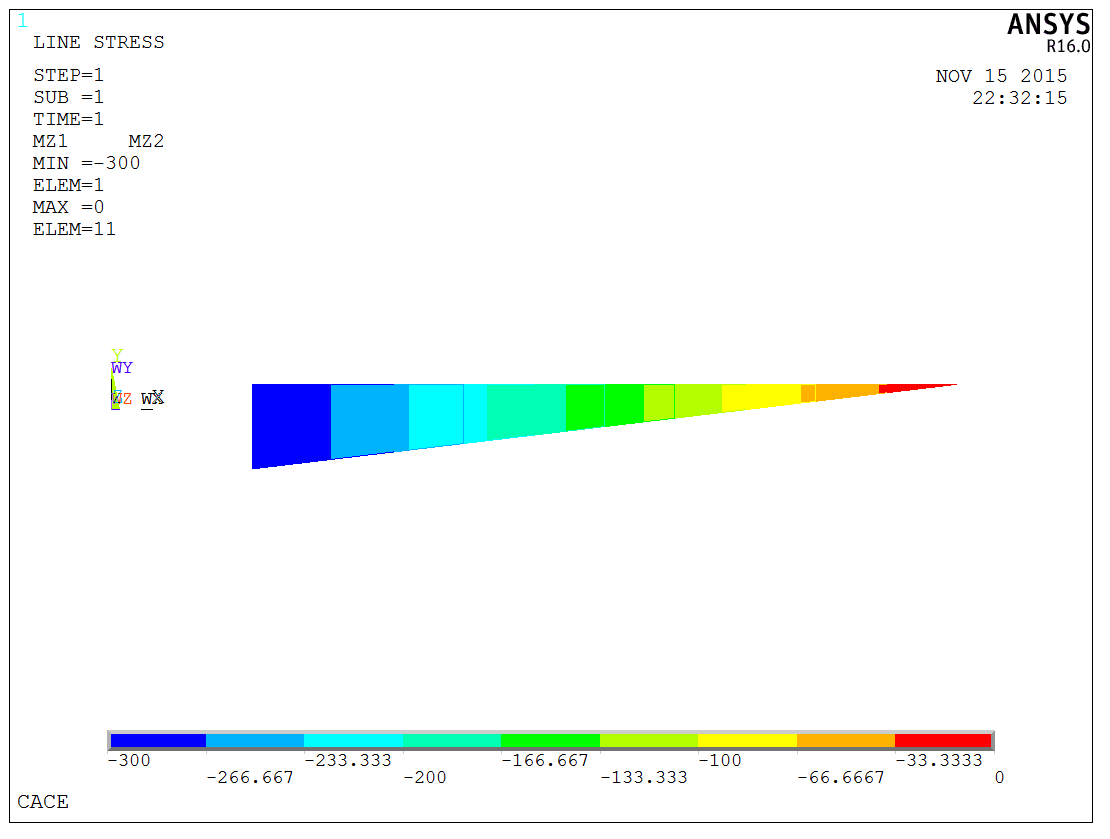# ANSYS约束方程的施加与分析

$Constant = \sum\limits_{I = 1}^N {\left( {Coeff\left( I \right) \times U\left( I \right)} \right)}$

CE, NEQN, CONST, NODE1, Lab1, C1, NODE2, Lab2, C2, NODE3, Lab3, C3

NEQN为约束方程编号，可取值为：

=N：任意编号；

=HIGH：既有约束方程的最高编号，这个非常适用于向已有的方程中增加自由度；

=NEXT：既有约束方程最高编号+1，自动编号选项；

CONST为方程的常数项Constant；

NODE1为约束方程的第一项的节点号，如果使用-NODE1则为删除该项；

Lab1为第一项的节点自由度标识符，可以用UX、UY、UZ、ROTx、ROTy、ROTz进行表示；

C1为第一项系数，如果设置为0则不计入该项。

$\frac{{U{X_2} – U{X_1}}}{{D{y_{2 – 1}}}} = – ROT{z_5}$

$0 = U{X_2} – U{X_1} + ROT{z_5} \times D{y_{2 – 1}}$

CE,1,0,2,UX,1,1,UX,-1,5,ROTZ,NY(2)-NY(1)APDL代码以如下所示：